Friday , May 24 2019
Home » Igcse Physics Revision Notes » Turning Effects Of Forces

# Turning Effects Of Forces

### Moments:

The moment of a force is the product of the force and the perpendicular distance from the pivot to the line of action of the force.

Moment of force =  Force × Distance

SI unit: Nm (Newton per metre)

It is a vector quantity as it has both magnitude and direction.

When more than one force acts in one direction, their overall turning effect is just the sum of their moments.

### Principal of a Moment:

For a system to be in equilibrium, the sum of the clockwise moment must be equal to the sum of the anti-clockwise moment.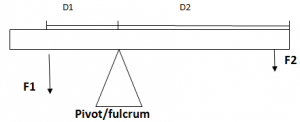Sum of anticlockwise moment =sum of clockwise moment

F1×D1=F2×D2

Conditions Of Equilibrium:

• The resultant force acting is zero or the forces are balanced.
• The resultant moment about the pivot is zero.

### Types of Equilibrium:

Un Stable Equilibrium: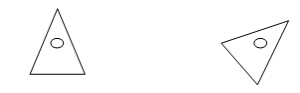Neutral Equilibrium: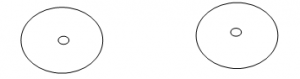### Centre Of Gravity:

Centre of gravity of an object is defined as the point through which weight appears to act.

It depends on the distribution of the mass.

The centre of gravity may also lie outside the object e.g. a ring

###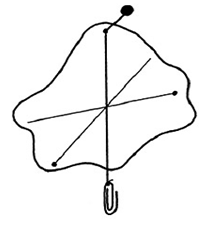• Make three holes near the edge of the lamina. The holes should be as far apart as possible from one another.
• Suspend the lamina through one of the holes using a pin.
• Hang a plumb line on the pin in front of the lamina.
• When the plumb line is steady draw a line on the lamina over the plumbline.
• Repeat the above for other two holes.
• The point of intersection of the three lines is the position of the centre of gravity.

### Precautions:

The holes should be small so that not too much of the lamina is removed.

The lamina should be free to swing about its point of suspension.

### Stability:

Stability refers to the ability of the object to regain its original position after it has been tilted slightly.

Stable equilibrium:

• Occurs when an object is placed in such a position that any disturbance effort would raise its centre of gravity.
• The centre of gravity will still fall in its base so it returns to its original position (as an anti clockwise moment is created as shown above by the arrowUnstable equilibrium

• Occurs when an object is placed in such a position that any disturbance effort would lower its centre of gravity
• The centre of gravity will no longer fall in its bade so it topples over to fall into a more stable position ( A clockwise moment is created shown by an arrow)Neutral equilibrium: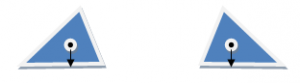• Neutral equilibrium occurs when an object is placed in such a position that any disturbance effort would not change the level of its centre of gravity.
• Thus, no such moment is created

### How to determine the stability of an object:

• The area of its base should as wide as possible.
• Centre of gravity should be as low as possible.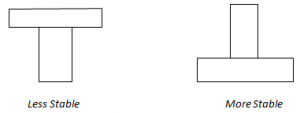### About Admin## Mass Weight And Density

Mass: Mass is the measure of amount of substance in a body. (Irrespective of its …

### 6 comments

1.please can we have the marking scheme for paper may june 2016 thnxx

2.Can you upload A-level Bio physics and chemistry may june 2016 papers Please?

3.thanks a lot! this is really helpful 😀